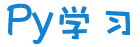注册    登录创作新主题

Python
 python开源   Django   Python   DjangoApp   pycharm
DATA
 docker   Elasticsearch

 问与答   闲聊   招聘   翻译   创业   分享发现   分享创造   求职   区块链   支付之战
aigc
 aigc   chatgpt
WEB开发
 linux   MongoDB   Redis   DATABASE   NGINX   其他Web框架   web工具   zookeeper   tornado   NoSql   Bootstrap   js   peewee   Git   bottle   IE   MQ   Jquery

Python88.com
 反馈   公告   社区推广

Py学习  »  chatgpt

# 陶哲轩：用 ChatGPT 写代码太省时间了

Python极客专栏 • 1 周前 • 12 次点击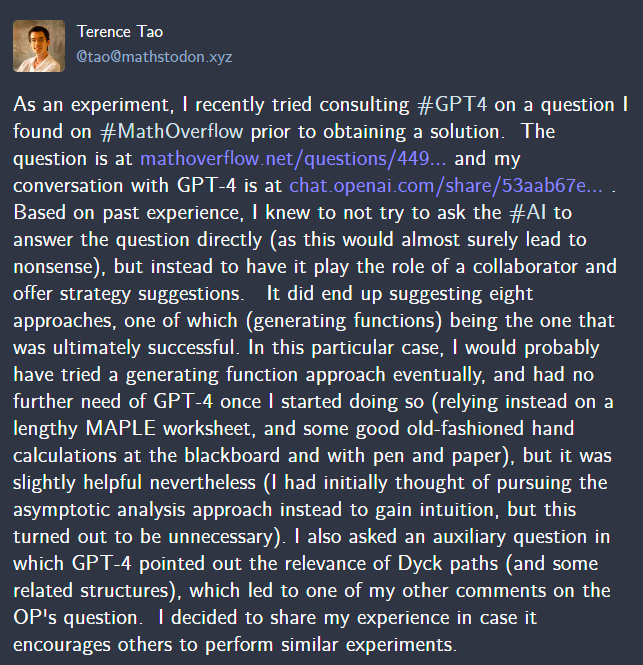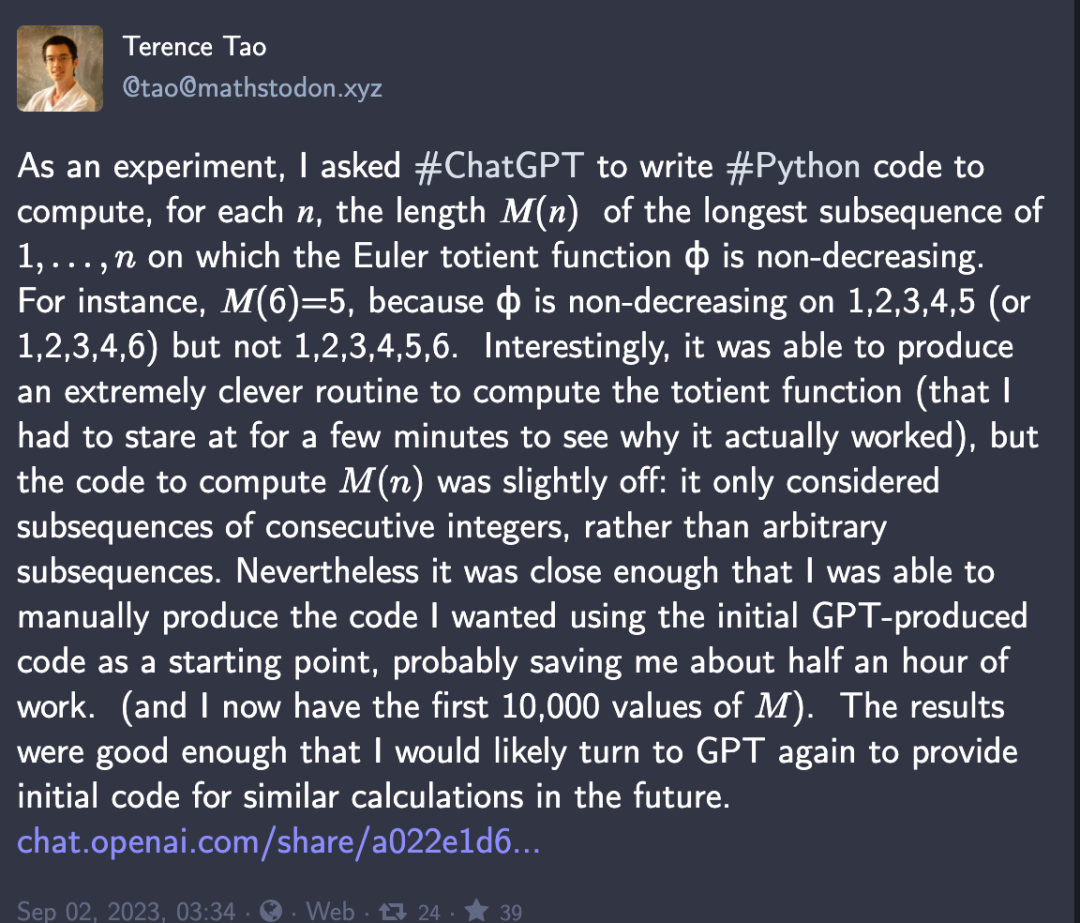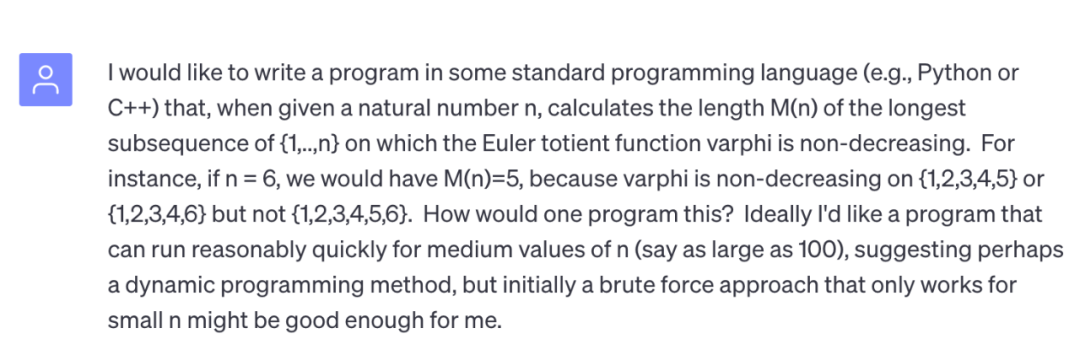``````import mathdef phi(n):    """Compute the Euler totient function for n."""    result = n    for i in range(2, int(math.sqrt(n)) + 1):        if n % i == 0:            while n % i == 0:                n //= i            result -= result // i    if n > 1:        result -= result // n    return resultdef is_non_decreasing(seq):    """Check if a sequence is non-decreasing."""    for i in range(1, len(seq)):        if seq[i] -1]:            return False    return Truedef longest_non_decreasing_phi_subsequence(n):    """Find the length of the longest subsequence of {1,...,n} on which phi is non-decreasing."""    phi_values = [phi(i) for i in range(1, n+1)]    max_length = 0    for i in range(n):        for j in range(i, n):            if is_non_decreasing(phi_values[i:j+1]):                max_length = max(max_length, j-i+1)    return max_lengthn = 6

print(longest_non_decreasing_phi_subsequence(n))  # Output: 5``````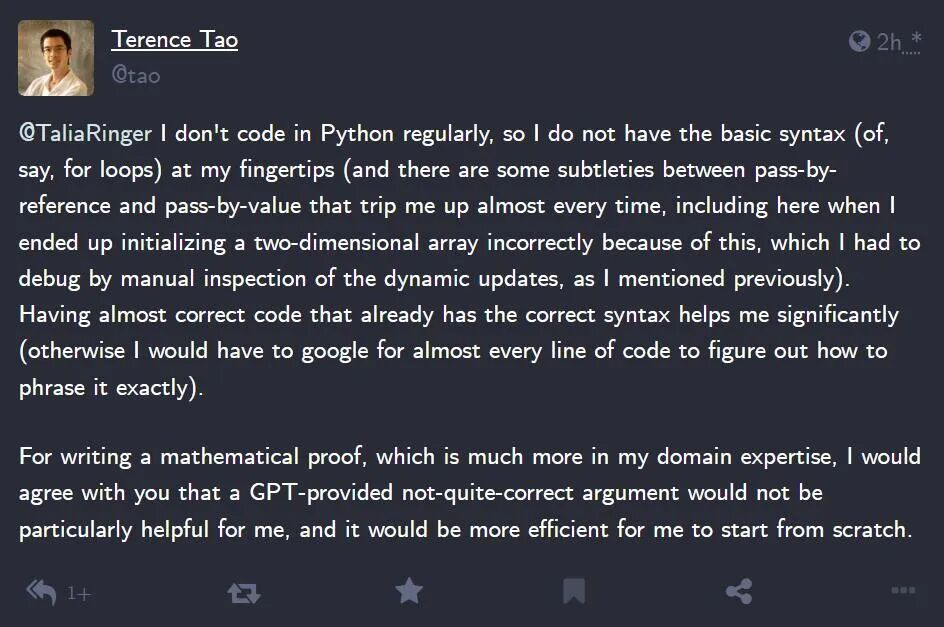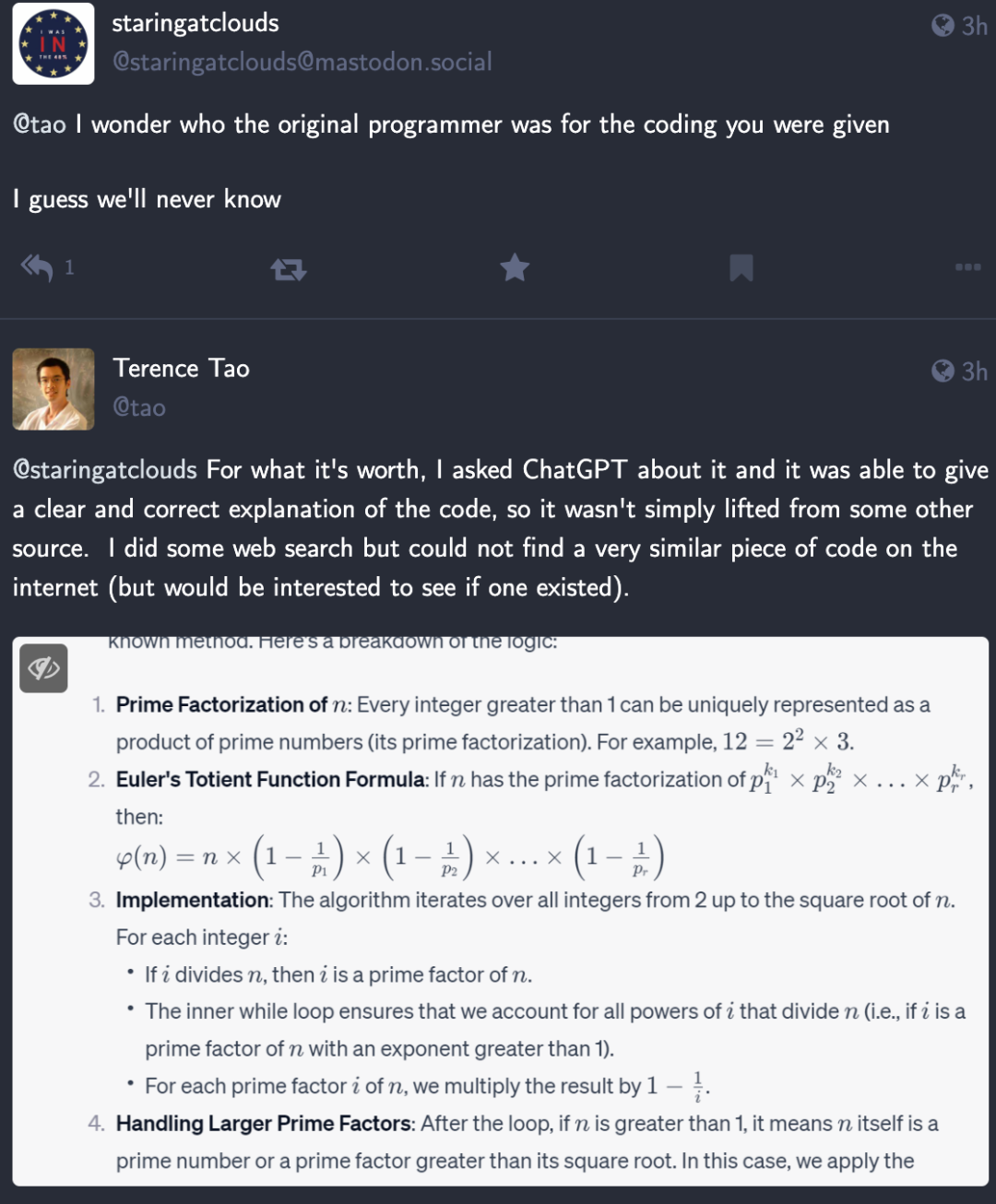https://mathstodon.xyz/@tao/110991566012143311

https://chat.openai.com/share/a022e1d6-dddc-4817-8bbd-944a3e742d9f

Python社区是高质量的Python/Django开发社区